Copyright (C) 2012-present, The Computational Data Science Lab
https://github.com/cdslaborg/paramonte

# Making 2D and 3D visualizations of the evolution of the proposal distributions of the Adaptive MCMC samplers of the ParaMonte library

NOTE
If you are viewing an HTML version of this MATLAB live script on the web, you can download the corresponding MATLAB live script visualization_proposal_ellipsoid.mlx file to this HTML page at,
https://github.com/cdslaborg/paramontex/tree/main/MATLAB/mlx
Once you download the file, open it in MATLAB to view and interact with its contents, which is the same as what you see on this page.
First, let's clean up the MATLAB environment and make sure the path to the ParaMonte library is in MATLAB's path list.
clc;
clear all;
close all;
format compact; format long;
%%%%%%%%%%%% IMPORTANT %%%%%%%%%%%%%
% Set the path to the ParaMonte library:
% Change the following path to the ParaMonte library root directory,
% otherwise, make sure the path to the ParaMonte library is already added
% to MATLAB's path list.
pmlibRootDir = './';
%%%%%%%%%%%%%%%%%%%%%%%%%%%%%%%%%%%%
% change MATLAB's working directory to the folder containing this script
% if MATLAB Live Scripts did not create a temporary folder, we would not
% have all of these problems!
try
setwdFilePath = websave("setwd.m","https://github.com/cdslaborg/paramontex/raw/main/MATLAB/mlx/setwd.m");
run(setwdFilePath); % This is a MATLAB script that you can download from the same GitHub location given in the above.
catch % alas, we will have to run the simulations in MATLAB Live Script's temporary folder
filePath = mfilename('fullpath');
[currentDir,fileName,fileExt] = fileparts(filePath); cd(currentDir);
cd(fileparts(mfilename('fullpath'))); % Change working directory to source code directory.
end

## ParaMonte's default visualization tools

The ParaMonte library ships with several visualization tools that automate much of the MATLAB coding required to visualize the output of the simulations performed by the ParMonte library samplers.
By replacing the input dataFrame to these tools and following the conventions of the ParaMonte library, one can also use these visualization tools for any dataset that may not have been generated by the ParaMonte library.
Consider the following restart file on the web which contains the restart information in ASCII format as indicated by the extension of the file (.txt). This file is generated by the ParaDRAM sampler for sampling a 4-dimensional Multivariate Normal distribution. Since this is a restart file as indicated by its suffix "_restart.txt" We will read this file via the ParaDRAM sampler's readRestart() method.
In addition to the restart information, the ASCII-formatted restart files that are generated by the Adaptive MCMC samplers of the ParaMonte library (such as the ParaDRAM and ParaDISE samplers) also contain information about the evolution of the adaptations of the proposal distributions of the MCMC samplers (if any adaptation occurs during the simulation). Monitoring this adaptation and ensuring that it diminishes toward the end of the simulation is not only essential for the successful unbiased sampling of the target density function but can also be educational.
For this reason, the adaptive MCMC samplers of the ParaMonte library provide a set of tools that can automatically visualize the evolution of the covariance or correlation matrices of the proposal distributions of the samplers. These tools are encapsulated in a class named EllipsoidPlot(). By default, when an ASCII-formated restart file is parsed, via for example, the readRestart() method of the ParaDRAM sampler, the parser routine automatically adds several plotting tools to the output object of the parser which contains the parsed restart data as well.
pm = paramonte();
Note that these visualization tools are not unique to this particular method of the ParaDRAM sampler or other ParaMonte samplers. For the sake of illustration however, we will create plots using the above dataset read via readrestart() method of the ParaDRAM routine.
restart = pmpd.restartList{1};
restart.plot.line.axes.kws.xscale = "log";
restart.plot.line.make();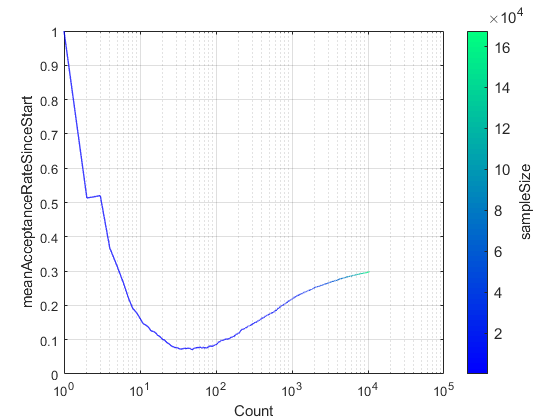Be default, the visualization tools are loaded with a set of predefined settings. For example, ParaMonte visualizations are by default colored. These however, can be readily changed. For example, to change the colormap,
restart.plot.line.colormap.values
ans = "winter"
restart.plot.line.colormap.values = autumn;
restart.plot.line.make()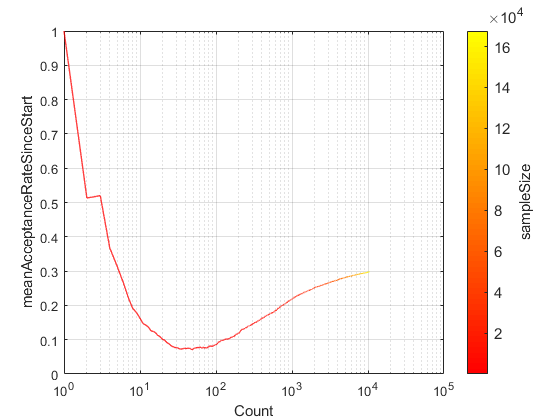More importantly, to visualize the evolution of the proposal distribution of the sampler in 2D via its covariance matrix, one can try,
restart.plot.covmat2.make();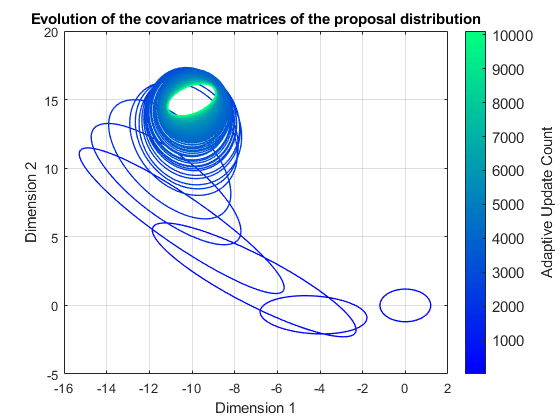Note that the covariance matrix of the proposal distribution is 4-dimensional in this example here since the target density function which has been sampled is 4 dimensional. However, only two dimensions out of the four dimensions of the covariance matrix can be visualized in a single plot. By default, the first two dimensions are selected to be visualized. However, this can be readily changed. For example, to visualize dimensions 2 and 4 of the covariance metrices of the proposal distribution,
restart.plot.covmat2.dimensionPair
ans = 1×2
1 2
restart.plot.covmat2.dimensionPair = [ 2 4 ];
restart.plot.covmat2.make();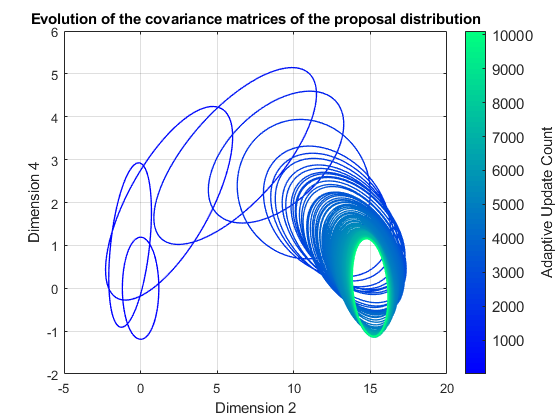By default, the covariance matrices are centered at the mean of the sampled stated by the adaptive MCMC sampler up to the stage when the covariance matrix was computed in the simulation. Sometimes, it helps to fix the centers of all covariance matrices all at the origin of the plot so that one could better focus on the variations in the shape and scale of the the covariance matrices. This can easily done,
restart.plot.covmat2.centerColumn
ans = 1×1 cell array {'meanVec'}
restart.plot.covmat2.centerColumn = []; % place all covariance matrices at the origin of the plot.
restart.plot.covmat2.make();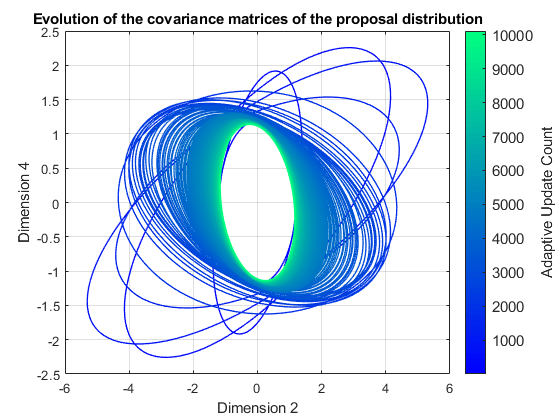The above plot is likely cluttered with too many ellipsoids. The number of ellipsoids can be reduced by only plotting a select representative number of ellipsoids form the restart file. Since much of the proposal adapations happen at the beginning of the simulation, a simple jump through the covariance matrices will likely not result in a good display,
restart.plot.covmat2.rows = 1:50:restart.count;
restart.plot.covmat2.make();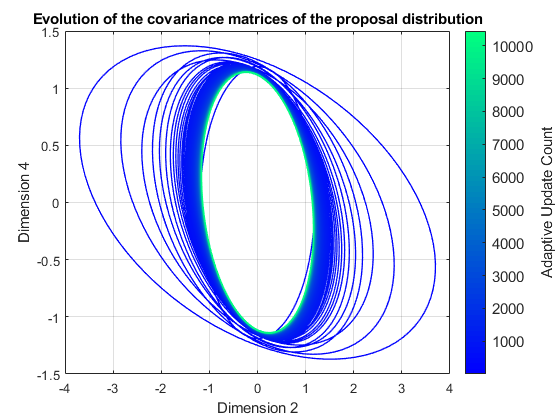A better approach would be to jump through the covariance matrices uniformly in the loarithmic space,
restart.plot.covmat2.rows = restart.plot.covmat2.getLogLinSpace ( 1.2 ... this is the base of the logarithm
, 0.7 ... this is the size of the jumps in the logarithmic space
... the start of the series goes here. The default is 1.
... the start of the series goes here. The default is the total number of covariance matrices.
);
restart.plot.covmat2.make();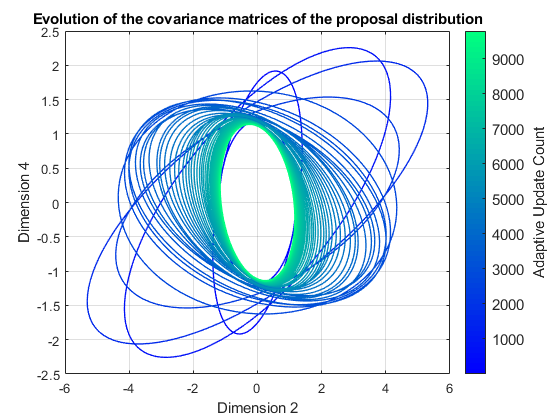To reset the properties of the plot object to the default settings, try,
restart.plot.covmat2.reset();
ParaDRAM - NOTE: resetting the properties of the covmat2 plot...
To reset the entire line object including reading the data again from the input dataFrame, try,
restart.plot.covmat2.reset("hard");
ParaDRAM - NOTE: creating the covmat2 plot object from scratch...

## Making 3D ellipsoid plots

If the 2D visualization is the optimal display one would wish to get, a 3D visualization may be more informative and illustrative,
restart.plot.covmat3.rows = restart.plot.covmat3.getLogLinSpace ( 1.3 ... this is the base of the logarithm
, 1 ... this is the size of the jumps in the logarithmic space
... the start of the series goes here. The default is 1.
... the start of the series goes here. The default is the total number of covariance matrices.
);
restart.plot.covmat3.make("dimensionPair", [1 2]);